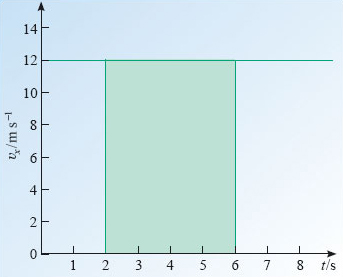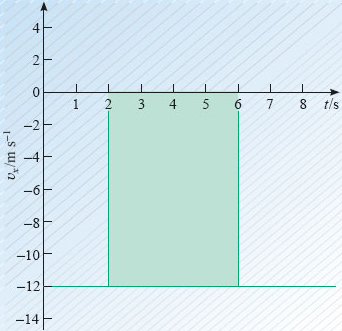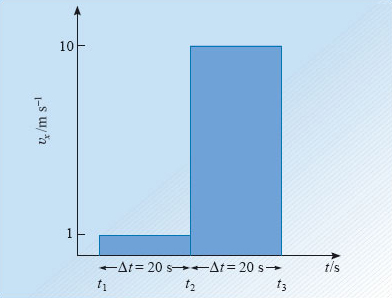Describing motion along a line

This free course is available to start right now. Review the full course description and key learning outcomes and create an account and enrol if you want a free statement of participation.

Free course

# 3.6 The signed area under a constant velocity-time graph

There is a simple feature of uniform velocity-time graphs that will be particularly useful to know about when we come to consider non-uniform motion in the next section. It concerns the relationship between the velocity-time graph and the change in position over a given time interval. Consider the following problem. A vehicle travels at a velocity vx = 12 m s−1 for 4 s. By how much does its position change over that interval?

The answer, from Equation 7, is 48 m. However, for our present purposes it is more instructive to work from the definition of uniform velocity (Equation 5), which may be rearranged by multiplying both sides of the equation by (t2t1) to give

x2x1 = vx (t2t1).

This tells us that the change in position during a given time interval is equal to the velocity multiplied by the time interval. So, a vehicle which travels at a constant velocity v﻿x = 12 m s﻿−1 over a time interval Δt = 4 s will change its position coordinate by Δx = 48 m.Figure 19: The area under a velocity-time graph

Now look at the velocity-time graph for this vehicle, which is given in Figure 19. The colour-shaded region corresponding to the 4 s interval between t = 2 s and t = 6 s is an example of an area under the graph: that is an area bounded by the plotted line, the horizontal axis and two vertical lines. The important point to note about this region is its area - not its physical area in terms of m2 of paper, but rather its area in terms of the units used on the axes of the graph. The area of a rectangle is the product of the lengths of two adjacent sides. So in Figure 19 the area under the graph is

vx × Δt = (12 m s−1) × (4 s) = 48 m.

Clearly, the area under the velocity-time graph, between the specified times, is exactly equal to the change in position coordinate between those times. In case you are worried that this relationship will break down if the velocity is negative, as in Figure 20, it should be added that areas that hang below the horizontal axis are conventionally regarded as negative areas. In this sense the area under a graph is a signed quantity that may be positive or negative; indeed, it is often referred to as the signed area under a graph.

Recognising the equality between the signed area under a uniform velocity-time graph and a change in position coordinate is not particularly helpful in itself, but it does pave the way for further developments, as does the next question.Figure 20: The area under a velocity-time graph is a signed quantity that may be negative

## Question 13

An athlete walks with a constant velocity v﻿x1 = 1 m s﻿−﻿1 for 20 s, and then abruptly starts running with a constant velocity v﻿﻿x﻿2 = 10 m s−﻿1, a velocity that is maintained for a further 20 s. What is the area under the corresponding velocity-time graph, and what physical interpretation could you give to that area?

### Answer

The relevant velocity-time graph is shown in Figure 21. In this case there are two rectangular areas to evaluate. Their total area is

(20 s) × (1 m s−1) + (20 s) × (10 m s−1) = 220 m.

This is equal to the change in position over the full 40 s duration of the motion.Figure 21: The graph for Question 13. Note that the time values are not known, only the durations, which have been indicated by Δt

Here we are using a double subscript on the symbol v. The subscript x denotes the x-direction as usual, and the 1 and 2 refer to the different stages of the motion.

S207_2

### Take your learning further

Making the decision to study can be a big step, which is why you'll want a trusted University. The Open University has 50 years’ experience delivering flexible learning and 170,000 students are studying with us right now. Take a look at all Open University courses.

If you are new to university level study, find out more about the types of qualifications we offer, including our entry level Access courses and Certificates.

Not ready for University study then browse over 900 free courses on OpenLearn and sign up to our newsletter to hear about new free courses as they are released.

Every year, thousands of students decide to study with The Open University. With over 120 qualifications, we’ve got the right course for you.

Request an Open University prospectus